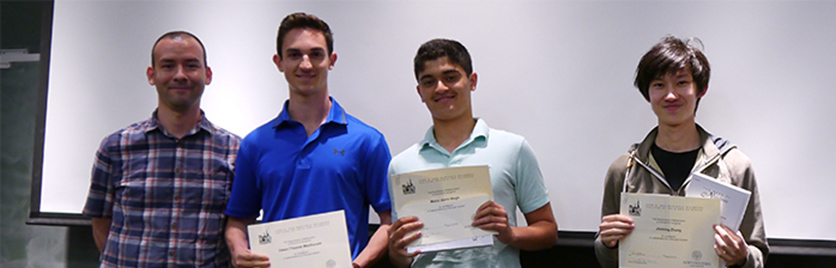# DEPARTMENT OF MATHEMATICS## SPS Mathematics Major Requirements

### The Mathematics Major

The mathematics major through the School of Professional Studies leads to a BPhil or BSGS degree.

• Basic courses
• MATH 220-A Single-Variable Differential Calculus
• MATH 220-B Single-Variable Integral Calculus
• MATH 226-CN Sequences and Series
• MATH 230-A Multivariable Differential Calculus
• MATH 230-B Multivariable Integral Calculus
• MATH 240-CN Linear Algebra
• Seven upper-level mathematics courses chosen from MATH 250-CN and mathematics courses numbered 300-CN and above